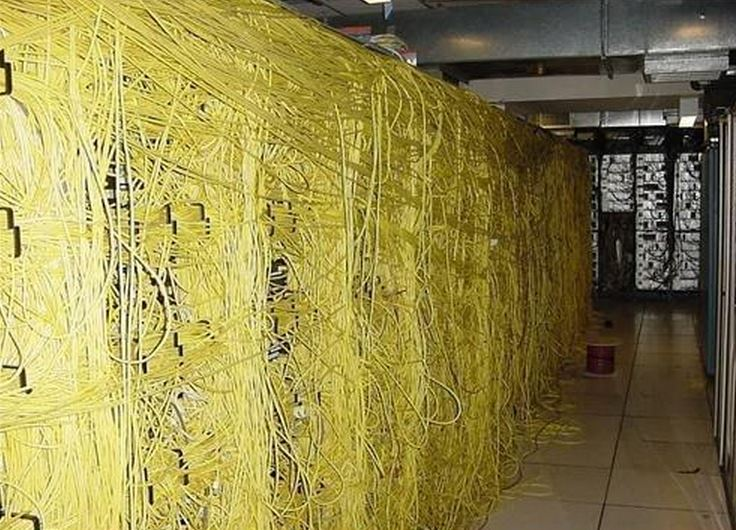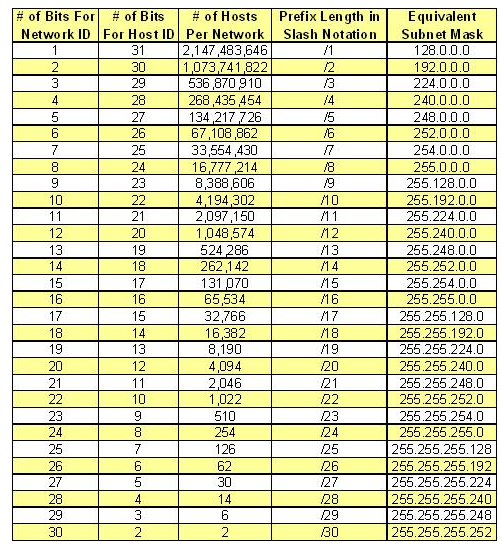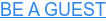### IP V4 Fundamentals

We all know, or should know that there are 3 primary classes of IP addresses we deal with Class A, B, and C. Each address has different ranges and as we move through the ranges

Address Class Range of First Octect Subnet Mask Network Bits Host Bits
Class A 0-127 255.0.0.0 8 24
Class B 128–191 255.255.0.0 16 16
Class C 192–223 255.255.255.0 24 8

If we are looking at an IP address we should be seeing bits, not decimals. Remember that an IP address has 4 octets (4 sets of 8 bits).

Each bit has a value going from the left to right. If a bit is 1 you add the value of that bit if it is 0 you obviously add nothing.

128 / 64 / 32 / 16 / 8 / 4 / 2 / 1

Thus 192.168.1.0 would be

128/ 64 . 128 / 32 / 8. 1/ . 0 If you add these values they equal 192.168.1.0

11000000.10101000.00000001.00000000

Try doing these IP address from Decimal to Binary

10.8.6.4

208.140.132.0

192.180.75.2

You cannot move on until you understand the basics above. You just can’t.

### Subnetting basics

First, we must understand that a Network bit is just what it sounds like a bit that represents the network. A Host bit is a bit that represents a host (PC, server, Interface, ect). When we subnet we take host bits and we assign them to networks so we can have multiple networks subnetted from a single main IP address.

So the first thing you need to understand is Classless Inter-Domain Routing (CIDR). I hate to say it but you really have to memorize these. If you memorize your CIDR’s it makes sub-netting so much faster (and easier). To that point, I have a CIDR List below.Ok, I am going to (at least I think I am going to) make sub-netting really easy.

There are 4 things you need to know with sub-netting (if you know these six things you will pass any Cisco sub-netting question on the ICND1).

1. Hosts= 2 to the number of host bits minus 2.
2. Networks= 2 to the number network bits
3. First Bit and Last bit of the range are reserved for the network and broadcast
5. You only go to /30 not /32 for networks
6. 255 means all bits in an octet are network bits

Ok so let’s say we get asked the following question. You have 10.1.1.2 255.255.255.252.

• What is the sub-network-  We know we are in the fourth octet so we take 256 and subtract 252 and that gives us a range of 4. Assuming subnet zero is allowed we begin at 10.1.1.0. We know our range is 10.1.1.0 – 10.1.1.3 (that's 4 addresses).
• What is the first and last usable address of the range- We know that the first address is the network and the last is the broadcast so we know the range is 10.1.1.1 -101.1.2.
• How many networks can we have- We have 30 network bits and we are sub-netting the 4th octet so we take 30-24 and that gives us 2 to the power of 6. Thus we have 64 potential networks.
• How many hosts can we have- We have 2 host bits 32-30. 2 to the power of 2 minus 2 is 2 so we can have 2 hosts.

Let’s do another

Say we get this address 192.168.1.54 /29

Well first, we memorized our CIDR table right? so then we know /29 means 255.255.255.248 ( a little trick is if you memorize /8 /16 /24 and /30 you can count down and up. For example /30 is 252 so we can say ok we know /29 is 248).

If so we know /29 means 255.255.255.248 ( a little trick is if you memorize /8 /16 /24 and /30 you can count down and up. For example /30 is 252 so we can say ok we know /29 is 248).

• What is the sub-network-  Well we know our multiple is 256-248 8. Now we know 40 is a multiple of 8 We start at 40 and count up. 40, 48, 56, 64… woh there we passed it. We know 54 is between 48 and 56 so we know our subnetwork is 192.168.1.48
• What is the first and last usable address of the range- Now we should cheat a little here, we should fill in the broadcast address first? If we know the broadcast is the last address of the range and the new range begins at 56 then we have a broadcast of 192.168.1.55 which means our address range must be192.168.1.49 – 192.168.1.54. (Remember we want the Address after the network and the address before the broadcast.
• What is the broadcast address We solved this in the previous question? 192.168.1.55
• How many networks can we have- We know we have 28 network bits we are a class C address and we are sub-netting the fourth octet. We take 29-24, we get 5 bits. 2 to the power of 5 is 32. So we have 32 networks.
• How many hosts can we have- We have 32 bits total so we take 32-29 which is 3. 2 to the power of 3 is 8 minus 2 (for our network and broadcast address) now we have 6 hosts.

Ok final example by this point you should be seeing how easy it is to subnet. It’s really not that hard, especially if someone like me can learn it

Well we know /18 is 255.255.192.0

Now things are going to get a little squirely but if you track with me you will understand this and it will make sense.

• What is the sub-network- Ok well we know we have 192 as our mask so let's do our math. 256-192 is 64 so we know we have multiples of 64 in the third octet. So if we are using classless or subnet-zero (remember those terms) we would start at 0. 0 , 64, 128 STOP! Ok, we know 16 is before 64 so our subnet must be 172.168.0.0
• What is the first and last usable address of the range- Ok things are a little weird here as we are dealing with two octets. We know 172.168.0.0 is our first address in the range so what is the last. Well if the next subnet is 172.168.64.0 then we know our last address is the number before that, 172.168.63.255. Now we know our last address is our broadcast so our range is 172.168.0.1 – 172.168.63.254
• How many networks can we have- So we know we have a /18 and we know Class B is a 16-bit mask by default we take 18-16 that gives us 2 and 2 to the power of 2 is 4 so we have 4 networks.
• How many hosts can we have- Alright now this will require a bit of math but we can do it. We take 32 minus 18 which is 14. Now we take 2 to the power of 14 which is 16384 we minus 2 and we get 16382 hosts.

Ok try out these addresses on your own. Feel free to print out the end of this blog post.

On the sub-network of 172.168.32.0 /19

• What is the first and last usable address of the range-
• How many networks can we have-
• How many hosts can we have-

• What is the sub-network-
• What is the first and last usable address of the range-
• How many networks can we have-
• How many hosts can we have-

• What is the sub-network-
• What is the first and last usable address of the range-
• How many networks can we have-
• How many hosts can we have-

### Recapping

Ok, I wanted to ensure I got sub-netting done because so many things I am writing about in my articles require a firm understanding of sub-netting. I wanted to ensure that those reading had a firm grasp on sub-netting.

I highly recommend Todd Lammel’s ICND1 book as my method was mostly developed off his methods.

Now that the article is done for today tell me your thoughts on the article? How could I improve the content? Does this help you learn?

Be sure to comment on the article and share with your friends.### Want to be a guest on the Podcast?